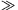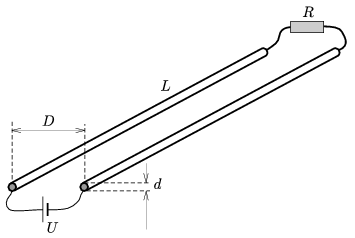Mathematical and Physical Journal
for High Schools
Issued by the MATFUND Foundation
 Already signed up? New to KöMaL?

#Problem P. 4550. (May 2013)

P. 4550. Two alike long, straight wires of diameter d, are at a distance of; their resistance is negligible and (LD). One ends of the wires are connected through a battery of voltage U, and the other ends of the wires are connected through a resistor of resistance R. What is the value of R if the electric and magnetic forces exerted between the wires are equal?(6 pont)

Deadline expired on June 10, 2013.

Sorry, the solution is available only in Hungarian. Google translation

Megoldásvázlat.

$\displaystyle R=\frac{1}{\pi} \sqrt{\frac{\mu_0}{\varepsilon_0}}\ln\frac{2D}{d}\approx 553\,\Omega.$

### Statistics:

 17 students sent a solution. 6 points: Antalicz Balázs, Balogh Menyhért, Kollarics Sándor, Kovács 444 Áron, Olosz Balázs. 5 points: Sárvári Péter, Szabó 928 Attila, Váli Tamás. 4 points: 1 student. 3 points: 4 students. 2 points: 1 student. 1 point: 1 student. 0 point: 2 students.

Problems in Physics of KöMaL, May 2013